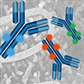# Product Results## Primary Antibody Conjugates

Choose from a wide range of directly conjugated primary antibodies, validated and optimized for your specific application. Not seeing the one you want? Let CST conjugate it for you.

# Product Name Application Reactivity
H, M, R
H, M, R
H, M, R
H
H
H, M
H, M
H, M, R, Mk
H, M, R, Mk
H, M, R, Mk
H, M, R, Mk
H, M, R, Mk
H, M, R, Mk
H, M, R, Mk
H, M, R, Mk
H, R
H, M, R, Mk
H, M, R, Mk
H
H
H
H
H
H
H, M, R, Mk, Z, Dg
H, M, R, Mk, Z, Dg
H
H
H, M, R
H, M, R, Mk